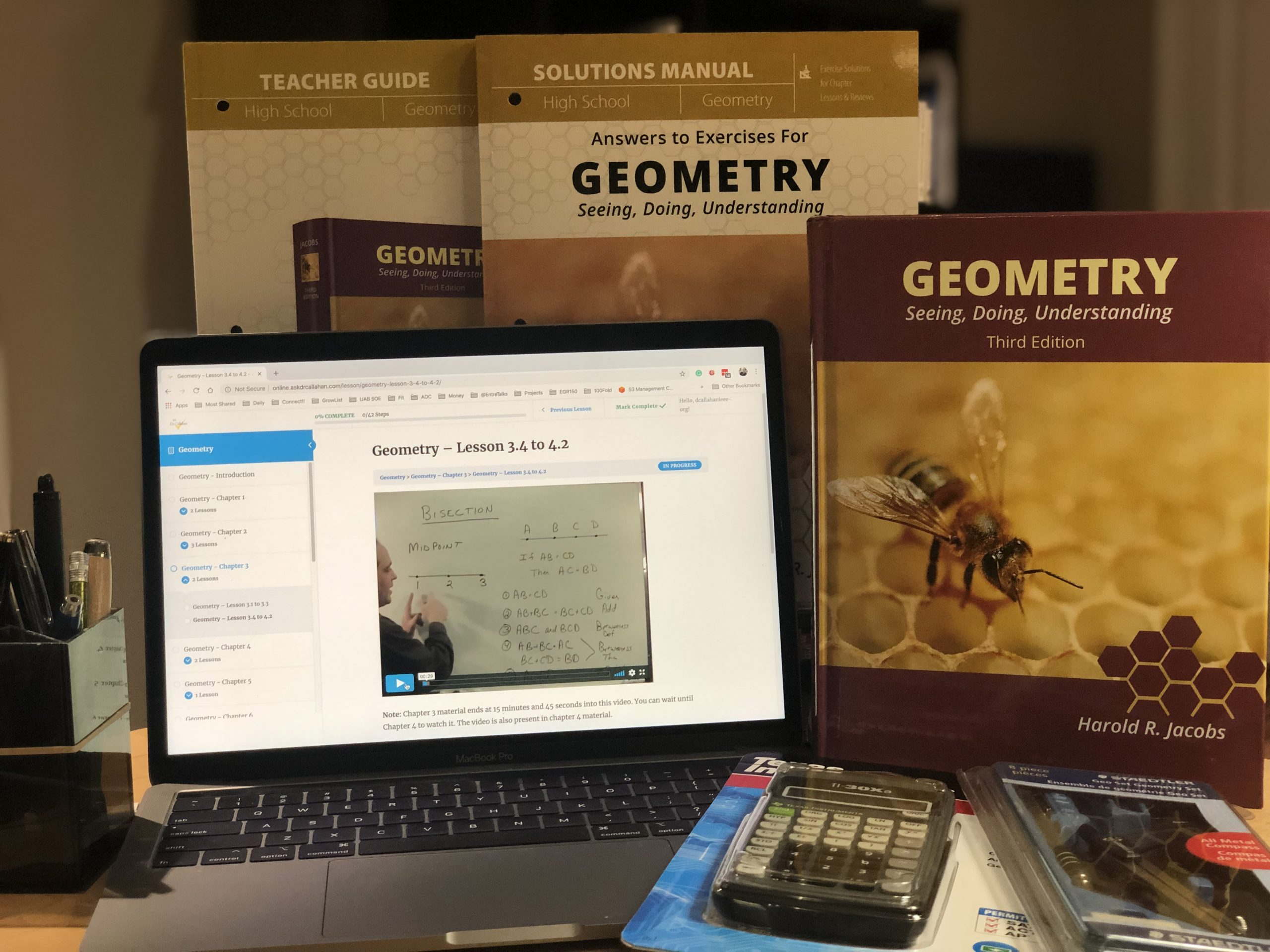Posted on

# Geometry Course Description for TranscriptIf you are looking for details on for a transcript for Jacobs Geometry Course, this description should do the trick.

Geometry is a branch of mathematics that deals with the understanding of the world around us by using measurement, properties, and relationships of points, lines, angles, surfaces, and solids. This course covers Euclidean geometry – which is a mathematical system where we assume a small set of truths (axioms) and from them, we deduce through logic, many other propositions (theorems).  This course emphasizes the concept of proofs, but in a reachable way that helps the student learn both the logic and the geometrical mathematics. Students who complete Geometry should take Algebra II with Trig (aka precalculus) next. Concepts from this course show up on the ACT and the SAT.

Topics Covered in this Course:

• Deductive Reasoning
• Lines and Angles
• Congruence
• Inequalities
• Parallel Lines